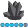## Proc Gplot ignoring symbol2

Hi,

I am interested in creating an individual trend plot for CD4 count over time per person with a regression line.The problem is that proc gplot ignores the symbol2 statement in my syntax and so the plot only has the individual trend lines and no regression line.

The code is below:

goptions reset=all;

proc gplot data=dataset;

plot cd4count*time=id/nolegend haxis=-1 to 300 by 10 vaxis=0 to 2000 by 100;

plot2 cd4count*time;

symbol1 v=none repeat=369 i=join color=red width=1;

symbol2 v=none i=r color=blue width=3;

label time='Months since seroconversion';

run;

I'll appreciate any help in fixing this issue.

Thanks

7 REPLIES 7

## Re: Proc Gplot ignoring symbol2

You need to fix the line style also.  Otherwise SAS will cycle through the line styles using SYMBOL1 before beginning to use SYMBOL2.

## Re: Proc Gplot ignoring symbol2

Thanks Tom. I have tried including the line style as shown below, but no luck.

goptions reset=all;

proc gplot data=dataset;

plot cd4count*time=id/nolegend haxis=-1 to 300 by 10 vaxis=0 to 2000 by 100;

plot2 cd4count*time;

symbol1 l=1 v=none repeat=369 i=join color=red width=1;

symbol2 l=2 v=none i=r color=blue width=3;

label time='Months since seroconversion';

run;

## Re: Proc Gplot ignoring symbol2

By any chance do you have an ID value that all of the  time values are outside 1 to 300 or cd4count outside 0 to 2000?

## Re: Proc Gplot ignoring symbol2

No, the ranges were selected to be within the minimum and maximum values obtained from proc means.ChrisNZ
Tourmaline | Level 20

## Re: Proc Gplot ignoring symbol2

I'd say your repeat= option is the culprit.

Remove it and if you get the plot2 start from there.

## Re: Proc Gplot ignoring symbol2

I have found it to be difficult (impossible?) to control the line symbols, when combining "plot y*x=z" with a "plot y*x", when doing a plot/plot2, or using the overlay option.

I would recommend restructuring your data so that you can plot them all using "plot y*x" (without the =z).  Or, you could annotate the regression line onto the plot.AncaTilea
Pyrite | Level 9

## Re: Proc Gplot ignoring symbol2

Using the SASHELP.CLASS data set as an example.

There are two genders (M and F) and age and weight as continuous.

In first plot, i want the age * weight by gender, so I use the symbol1 i = join and repeat = 2(for my 2 genders), so for you repeat = 369 I assume is for the total number of IDs.

Then I do the plot2, for the regression line (overall) between age and weight, using the symbol2 statement with i = r.

So, the only difference between your code and mine is where I have the symbol statement (immediately after the corresponding plot statement).(which should not matter)

goptions reset=all;

proc sort data = sashelp.class out = temp;by weight;

proc gplot data = temp;

plot age*weight = sex;

symbol1 v = dot repeat = 2 i = join color = blue width = 3;

plot2 age*weight;

symbol2 v = none i = r color = green width = 2;

run;quit;

Would you be able to provide a sample data?

Discussion stats
• 7 replies
• 1300 views
• 0 likes
• 6 in conversation